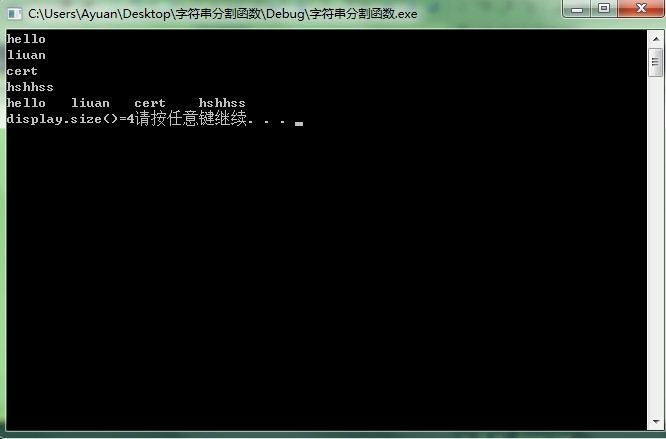利用容器来拆分字符串

#include<iostream>
#include <vector>
#include <string>
#include <cctype>
using namespace  std;
vector<string> division_word(const string &str);
int main()
{
string str="hello liuan cert    hshhss";

vector<string> display;
display=division_word(str);    //容器display 保存分割后的字符串
//size_type 是保存容器大小的变量
for ( vector<string>::size_type i=0;i !=display.size();++i )
{
cout<<display.at(i)<<endl;
}
vector<string>::iterator iter=display.begin();//定义一个string 型的迭代器，指向display的首地址  注意迭代器的类型和容器的类型匹配，迭代器的实质是指针，但是比指针使用更加安全
for(;iter!=display.end();iter++)
{
cout<<(*iter)<<"/t";   //利用迭代器把容器遍历
}
cout<<endl;
cout<<"display.size()="<<display.size();
system("pause");
return 0;

}

vector<string> division_word(const string &str)
{
vector<string> temp;   //临时容器
typedef string::size_type string_size; //string::size_type 是一个typtdef，其实就是size_t 用来保存其长度
string_size i=0;
while (i!=str.size())
{
//检测空白符，找到第一个不是空白符的字符
while(i !=str.size() && isspace(str[i]))  //
++i;
string_size j=i;
//从i查找下一个空白符
while(j !=str.size() && !isspace(str[j]) )//isspace(int c)  判断c是否是一个空字符 空返回非零即 1  非空返回0
++j;
if (i!=j)    //j最后是下一个非空字符位置
{
/*如果两者 [i,j)区域存在的话，
把从i索引开始的j-i个字符也就是单词复制到temp中
在继续查找

public String substring(int beginIndex, int endIndex)返回一个新字符串，它是此字符串的一个子字符串。
该子字符串从指定的 beginIndex 处开始，一直到索引 endIndex - 1 处的字符。因此，该子字符串的长度为 endIndex-beginIndex。 */
temp.push_back(str.substr(i,j-i));     //将该字符串压入vector
i=j;
}
}
return temp;
}

"unhappy".substring(2) returns "happy"

isspace(int _c)参数是int型的，如果传入的是char型的，会利用ASCII码转换

vector是C++标准模板库的内容

vector<int> test;//建立一个vector

test.pushback(1);//把1和2压入vector 这样test就是1,test就是2

test.pushback(2);